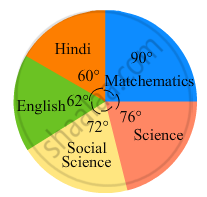Share

# In the Following Figure, the Pie-chart Shows the Marks Obtained by a Student in Various Subjects. If the Student Scored 135 Marks in Mathematics, Find the Total Marks in All the Subjects. - Mathematics

Course
ConceptIntroduction of Circle Graph Or Pie Chart

#### Question

In the following figure, the pie-chart shows the marks obtained by a student in various subjects. If the student scored 135 marks in mathematics, find the total marks in all the subjects. Also, find his score in individual subjects.#### Solution

$\text{ Marks scored in mathematics }= \frac{\text{ Central angle of corresponding sector }\times\text{ Total Marks }}{360^\circ}$
$135 = \frac{90^\circ \times \text{ Total }}{360^\circ}$
Total Marks = 540

Marks scored in Hindi = (Central angle of Hindi x Total)/360
= (60 x 540)/360 marks = 90 marks
Similarly, marks scored in science = (76 x 540) /360 marks = 114 marks
Marks scored in social science = (72 x 540) /360 marks = 108 marks
Marks scored in English = (62 x 540)/360 marks = 93 marks

Is there an error in this question or solution?

#### APPEARS IN

RD Sharma Solution for Mathematics for Class 8 by R D Sharma (2019-2020 Session) (2017 to Current)
Chapter 25: Data Handling-III (Pictorial Representation of Data as Pie Charts or Circle Graphs)
Ex. 25.2 | Q: 5 | Page no. 22

#### Video TutorialsVIEW ALL 

Solution In the Following Figure, the Pie-chart Shows the Marks Obtained by a Student in Various Subjects. If the Student Scored 135 Marks in Mathematics, Find the Total Marks in All the Subjects. Concept: Introduction of Circle Graph Or Pie Chart.
S